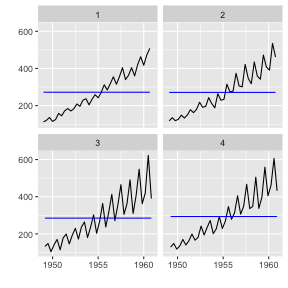This document explains time series related plotting using `ggplot2` and `{ggfortify}`.

# Plotting ts objects

`{ggfortify}` let `{ggplot2}` know how to interpret `ts` objects. After loading `{ggfortify}`, you can use `ggplot2::autoplot` function for `ts` objects.

``````library(ggfortify)
autoplot(AirPassengers)
``````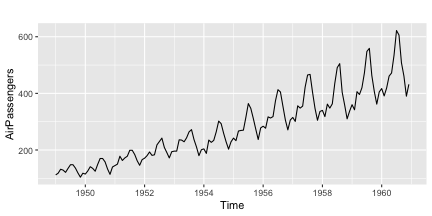To change line colour and line type, use `ts.colour` and `ts.linetype` options. Use `help(autoplot.ts)` (or `help(autoplot.*)` for any other objects) to check available options.

``````autoplot(AirPassengers, ts.colour = 'red', ts.linetype = 'dashed')
``````Multivariate time series will be drawn with facets.

``````library(vars)
``````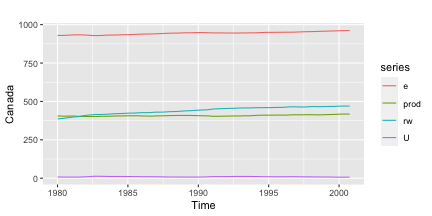Specify `facets = FALSE` to draw on single axes.

``````autoplot(Canada, facets = FALSE)
``````Also, `autoplot` can handle other time-series-likes. Supported packages are:

• `zoo::zooreg`
• `xts::xts`
• `timeSeries::timSeries`
• `tseries::irts`
``````library(xts)
autoplot(as.xts(AirPassengers), ts.colour = 'green')

library(timeSeries)
autoplot(as.timeSeries(AirPassengers), ts.colour = ('dodgerblue3'))
``````

# Specifying geometrics

You can change `{ggplot2}` geometrics specifying by its name. Geometrics currently supported are `line`, `bar`, `ribbon` and `point`.

``````autoplot(AirPassengers, ts.geom = 'bar', fill = 'blue')
````````````autoplot(AirPassengers, ts.geom = 'ribbon', fill = 'green')
````````````autoplot(AirPassengers, ts.geom = 'point', shape = 3)
``````As described above, multivariate timeseries can be drawn in a single grid specifying `facets = FALSE`. Time series are not stacked by default. Specifying `stacked = TRUE` allows stacking.

``````mts <- ts(data.frame(a = c(1, 2, 3, 4, 4, 3), b = c(3, 2, 3, 2, 2, 1)), start = 2010)
autoplot(mts, ts.geom = 'bar', facets = FALSE)
``````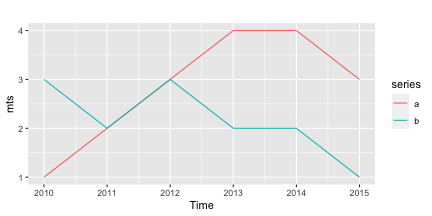``````autoplot(mts, ts.geom = 'bar', facets = FALSE, stacked = TRUE)
````````````autoplot(mts, ts.geom = 'ribbon', facets = FALSE)
````````````autoplot(mts, ts.geom = 'ribbon', facets = FALSE, stacked = TRUE)
``````# Plotting with forecast package

`{ggfortify}` supports `forecast` object in the `{forecast}` package.

``````library(forecast)
d.arima <- auto.arima(AirPassengers)
d.forecast <- forecast(d.arima, level = c(95), h = 50)
autoplot(d.forecast)
``````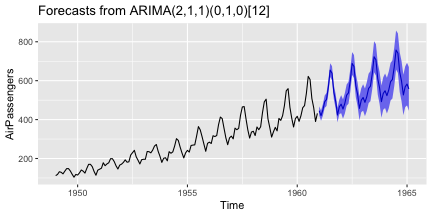There are some options to change basic settings.

``````autoplot(d.forecast, ts.colour = 'firebrick1', predict.colour = 'red',
predict.linetype = 'dashed', conf.int = FALSE)
``````# Plotting with vars package

`ggfortify` supports `varpred` object in `vars` package.

``````library(vars)
d.vselect <- VARselect(Canada, lag.max = 5, type = 'const')\$selection
d.var <- VAR(Canada, p = d.vselect, type = 'const')
``````

Available options are the same as `forecast`.

``````autoplot(predict(d.var, n.ahead = 50), ts.colour = 'dodgerblue4',
predict.colour = 'blue', predict.linetype = 'dashed')
``````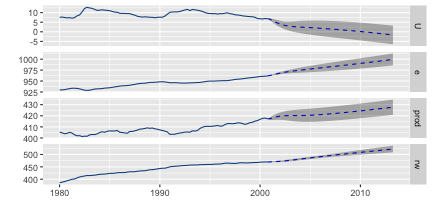# Plotting with changepoint package

`{ggfortify, eval=hasDep}` supports `cpt` object in `{changepoint}` package.

``````library(changepoint)
autoplot(cpt.meanvar(AirPassengers))
``````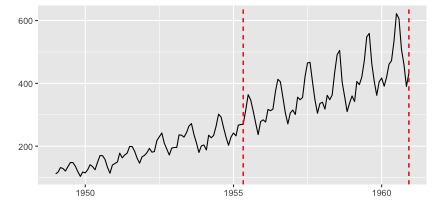You can change some options for `cpt`.

``````autoplot(cpt.meanvar(AirPassengers), cpt.colour = 'blue', cpt.linetype = 'solid')
``````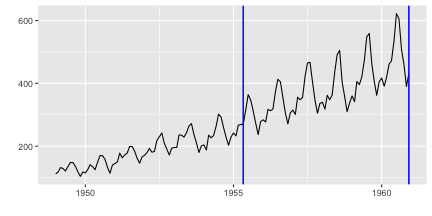# Plotting with strucchange package

`ggfortify` supports `breakpoints` object in `strucchange` package. Same plotting options as `changepoint` are available.

``````library(strucchange)
autoplot(breakpoints(Nile ~ 1), ts.colour = 'blue', ts.linetype = 'dashed',
cpt.colour = 'dodgerblue3', cpt.linetype = 'solid')
``````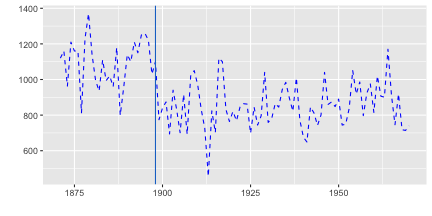# Plotting with `{KFAS}` package

You can use `autoplot` in almost the same manner as `{dlm}`. Note that `autoplot` draws smoothed result if it exists in `KFAS::KFS` instance, and `KFAS::KFS` contains smoothed result by default.

``````library(KFAS)
model <- SSModel(
Nile ~ SSMtrend(degree=1, Q=matrix(NA)), H=matrix(NA)
)

fit <- fitSSM(model=model, inits=c(log(var(Nile)),log(var(Nile))), method="BFGS")
smoothed <- KFS(fit\$model)
autoplot(smoothed)
``````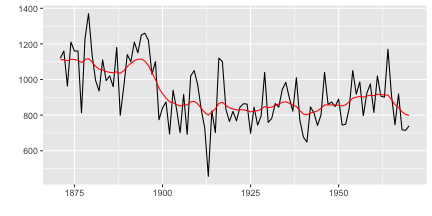If you want filtered result, specify `smoothing='none'` when calling `KFS`. For details, see `help(KFS)`.

``````filtered <- KFS(fit\$model, filtering="mean", smoothing='none')
autoplot(filtered)
``````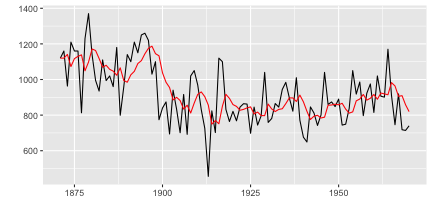Also, `KFAS::signal` will retrieve specific state from `KFAS::KFS` instance. The result will be a `list` which contains the retrieved state as `ts` in `signal` attribute. `ggfortify` can autoplot it using class inference.

``````trend <- signal(smoothed, states="trend")
class(trend)
``````
``````##  "list"
``````

Because `signal` is a `ts` instance, you can use `autoplot` and `p` option as the same as `dlm::dlmSmooth` example.

``````p <- autoplot(filtered)
autoplot(trend, ts.colour = 'blue', p = p)
``````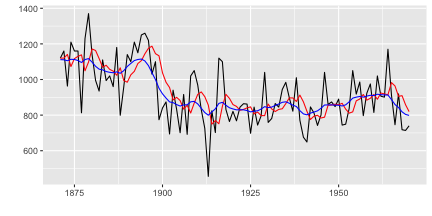# Plotting time series statistics

`{ggfortify}` supports following time series related statistics in `stats` package:

• `stl`, `decomposed.ts`
• `acf`, `pacf`, `ccf`
• `spec.ar`, `spec.pgram`
• `cpgram` (covered by `ggcpgram`)
``````autoplot(stl(AirPassengers, s.window = 'periodic'), ts.colour = 'blue')
``````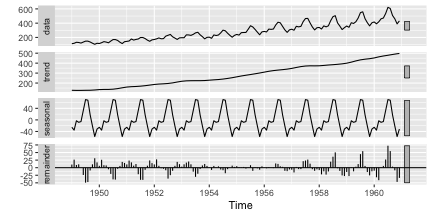NOTE With `acf` and `spec.*`, specify `plot = FALSE` to suppress default plotting outputs.

``````autoplot(acf(AirPassengers, plot = FALSE))
``````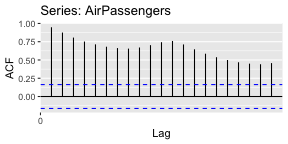You can pass some options when plotting `acf` via `autoplot`. Built-in `acf` calcurates the confidence interval at plotting time and doesn’t hold the result, equivalent options can be passed to `autoplot`. Following example shows to change the value of confidence interval and method (use `ma` assuming the input follows MA model).

``````autoplot(acf(AirPassengers, plot = FALSE), conf.int.fill = '#0000FF', conf.int.value = 0.8, conf.int.type = 'ma')
````````````autoplot(spec.ar(AirPassengers, plot = FALSE))
``````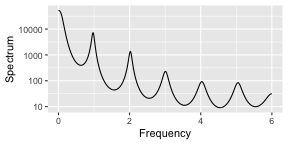`ggcpgram` should output the cumulative periodogram as the same as `cpgram`. Because `cpgram` doesn’t have return value, we cannot use `autoplot(cpgram(...))`.

``````ggcpgram(arima.sim(list(ar = c(0.7, -0.5)), n = 50))
``````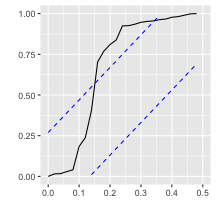`ggtsdiag` should output the similar diagram as `tsdiag`.

``````library(forecast)
ggtsdiag(auto.arima(AirPassengers))
``````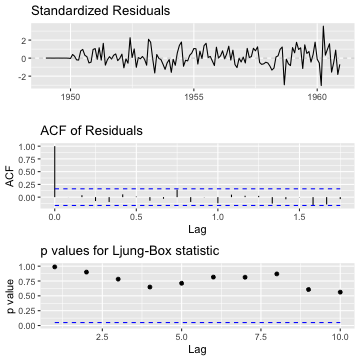`ggfreqplot` is a genelarized `month.plot`. You can pass `freq` if you want, otherwise time-series’s frequency will be used.

``````ggfreqplot(AirPassengers)
``````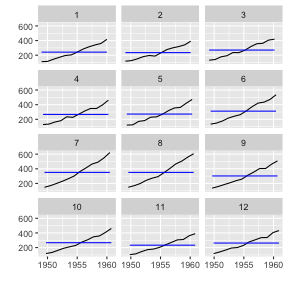``````ggfreqplot(AirPassengers, freq = 4)
``````